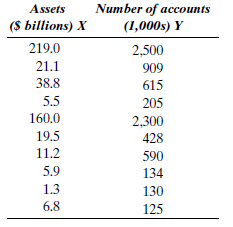# Business Forecasting Study Set 1

## Quiz 6 : Multiple Regression AnalysisLooking for Introduction To Business Homework Help?

## Quiz 6 :Multiple Regression Analysis

Question TypeWhat are the characteristics of a good predictor variable?
Free
Essay

The predictor variable is indicated as highly correlated with the response variable (Independent variable). However, these variables are not highly correlated with the other predictor variables. Hence, this is the characteristics of a good predictor variable.

TagsWhat are the assumptions associated with the multiple regression model?
Free
Essay

The four regression model assumptions are as follows:
• Zero mean assumption
• Constant variance assumption
• Independence assumption
• Normality assumption
Zero Mean Assumption:
The mean of the residual values is zero. That is, the residuals are independent with each other.
Constant variance assumption:
The variance of the values of independent variables is constant.
Independence assumption:
There is a linear relation between the response variable and each predictor variable.
Normality assumption:
The residual values are distributed as normal in the formation of the regression equation.

TagsWhat does the partial, or net, regression coefficient measure in multiple regression?
Free
Essay

The partial, or net regression coefficient is used to measure the average change (increase or decrease) in the response variable as per unit changes (increase or decrease) in the corresponding predictor variable, considering other predictor variables as constant.

TagsWhat does the standard error of the estimate measure in multiple regression?
Essay
TagsYour estimated multiple regression equation is ? = 7.52 + 3 X 1 ? 12.2 X 2. Predict the value of Y if X 1 = 20 and X 2 = 7.
Essay
TagsExplain each of the following concepts: a. Correlation matrix b. R 2 c. Multicollinearity d. Residual e. Dummy variable f. Stepwise regression
Essay
TagsMost computer solutions for multiple regression begin with a correlation matrix. Examining this matrix is often the first step when analyzing a regression problem that involves more than one independent variable. Answer the following questions concerning the correlation matrix given in Table P-7. a. Why are all the entries on the main diagonal equal to 1.00? b. Why is the bottom half of the matrix below the main diagonal blank? c. If variable 1 is the dependent variable, which independent variables have the highest degree of linear association with variable 1? d. What kind of association exists between variables 1 and 4? e. Does this correlation matrix show any evidence of multicollinearity? f. In your opinion, which variable or variables will be included in the best forecasting model? Explain. g. If the data given in this correlation matrix are run on a stepwise program, which independent variable (2, 3, 4, 5, or 6) will be the first to enter the regression function? TABLE P-7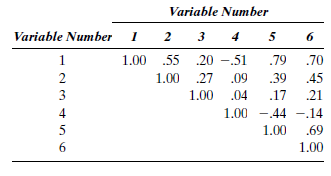Essay
TagsJennifer Dahl, supervisor of the Circle O discount chain, would like to forecast the time it takes to check out a customer. She decides to use the following independent variables: number of purchased items and total amount of the purchase. She collects data for a sample of 18 customers, shown in Table P-8. a. Determine the best regression equation. b. When an additional item is purchased, what is the average increase in the checkout time? c. Compute the residual for customer 18. d. Compute the standard error of the estimate. e. Interpret part d in terms of the variables used in this problem. f. Compute a forecast of the checkout time if a customer purchases 14 items that amount to $70. g. Compute a 95% interval forecast for your prediction in part f. h. What should Jennifer conclude? TABLE P-8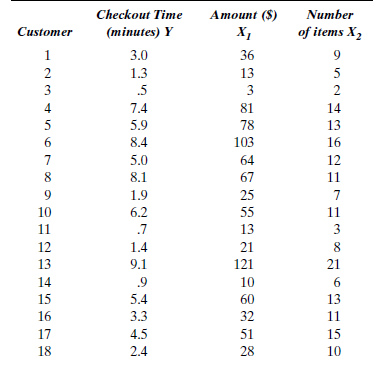Essay Answer: TagsTable P-9 contains data on food expenditures, annual income, and family size for a sample of 10 families. a. Construct the correlation matrix for the three variables in Table P-9. Interpret the correlations in the matrix. b. Fit a multiple regression model relating food expenditures to income and family size. Interpret the partial regression coefficients of income and family size. Do they make sense? c. Compute the variance inflation factors ( VIFs ) for the independent variables. Is multicollinearity a problem for these data? If so, how might you modify the regression model? TABLE P-9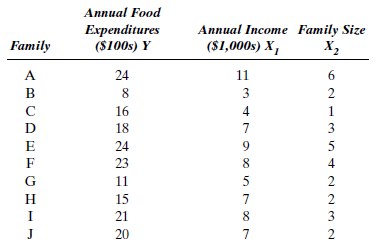Essay Answer: TagsBeer sales at the Shapiro One-Stop Store are analyzed using temperature and number of people (age 21 or over) on the street as independent variables. A random sample of 20 days is selected, and the following variables are measured: Y = the number of six-packs of beer sold each day X 1 = the daily high temperature X 2 = the daily traffic count The data are analyzed using multiple regression. The partial computer output is in Table P-10. a. Analyze the correlation matrix. b. Test the hypothesis H 0 : ? j = 0, j = 1.2, at the.01 significance level. c. Forecast the volume of beer sold if the high temperature is 60 degrees and the traffic count is 500 people. d. Calculate R 2 , and interpret its meaning in terms of this problem. e. Calculate the standard error of the estimate. f. Explain how beer sales are affected by an increase of one degree in the high temperature. g. State your conclusions for this analysis concerning the accuracy of the forecasting equation and also the contributions of the independent variables. TABLE P-10 Minitab Output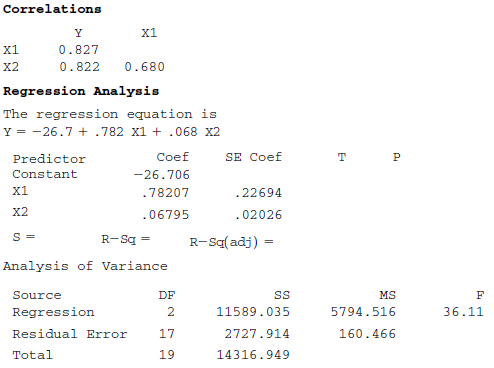Essay Answer: TagsA taxi company is interested in the relationship between mileage, measured in miles per gallon, and the age of cars in its fleet. The 12 fleet cars are the same make and size and are in good operating condition as a result of regular maintenance. The company employs both male and female drivers, and it is believed that some of the variability in mileage may be due to differences in driving techniques between the groups of drivers of opposite gender. In fact, other things being equal, women tend to get better mileage than men. Data are generated by randomly assigning the 12 cars to five female and seven male drivers and computing miles per gallon after 300 miles. The data appear in Table P-11. a. Construct a scatter diagram with Y as the vertical axis and X 1 as the horizontal axis. Identify the points corresponding to male and female drivers, respectively. b. Fit the regression model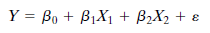and interpret the least squares coefficient, b 2. c. Compute the fitted values for each of the ( X 1 , X 2 ) pairs, and plot the fitted values on the scatter diagram. Draw straight lines through the fitted values for male drivers and female drivers, respectively. Specify the equations for these two straight lines. d. Suppose gender is ignored. Fit the simple linear regression model, Y= ? 0 + ? 1 X 1 + ? , and plot the fitted straight line on the scatter diagram. Is it important to include the effects of gender in this case? Explain. TABLE P-11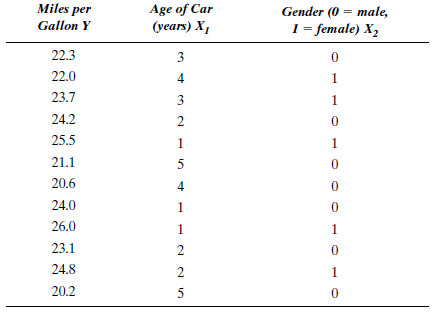Essay Answer: TagsThe sales manager of a large automotive parts distributor, Hartman Auto Supplies, wants to develop a model to forecast as early as May the total annual sales of a region. If regional sales can be forecast, then the total sales for the company can be forecast. The number of retail outlets in the region stocking the company's parts and the number of automobiles registered for each region as of May 1 are the two independent variables investigated. The data appear in Table P-12. a. Analyze the correlation matrix. b. How much error is involved in the prediction for region 1? c. Forecast the annual sales for region 12, given 2,500 retail outlets and 20.2 million automobiles registered. d. Discuss the accuracy of the forecast made in part c. e. Show how the standard error of the estimate was computed. f. Give an interpretation of the partial regression coefficients. Are these regression coefficients sensible? g. How can this regression equation be improved? TABLE P-12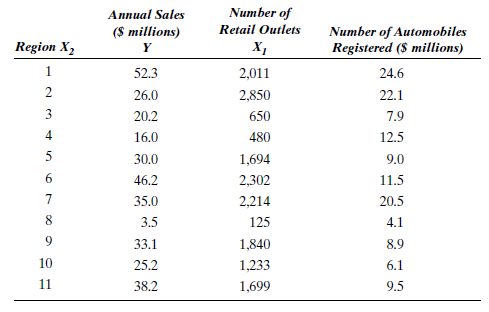Essay Answer: TagsThe sales manager of Hartman Auto Supplies decides to investigate a new independent variable, personal income by region (see Problem 12). The data for this new variable are presented in Table P-13. a. Does personal income by region make a contribution to the forecasting of sales? b. Forecast annual sales for region 12 for personal income of$40 billion and the values for retail outlets and automobiles registered given in part c of Problem 12. c. Discuss the accuracy of the forecast made in part b. d. Which independent variables would you include in your final forecast model? Why? TABLE P-13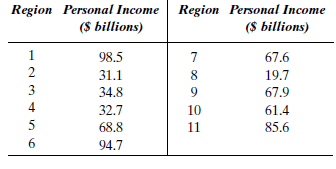Essay
TagsThe Nelson Corporation decides to develop a multiple regression equation to forecast sales performance. A random sample of 14 salespeople is interviewed and given an aptitude test. Also, an index of effort expended is calculated for each salesperson on the basis of a ratio of the mileage on his or her company car to the total mileage projected for adequate coverage of territory. Regression analysis yields the following results: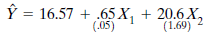The quantities in parentheses are the standard errors of the partial regression coefficients. The standard error of the estimate is 3.56. The standard deviation of the sales variable is s y = 16.57. The variables are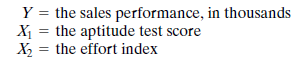a. Are the partial regression coefficients significantly different from zero at the.01 significance level? b. Interpret the partial regression coefficient for the effort index. c. Forecast the sales performance for a salesperson who has an aptitude test score of 75 and an effort index of.5. d. Calculate the sum of squared residuals, ?( Y ? ? ) 2. e. Calculate the total sum of squares,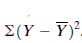. f. Calculate R 2 , and interpret this number in terms of this problem. g. Calculate the adjusted coefficient of determination,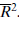Essay
TagsWe might expect credit card purchases to differ from cash purchases at the same store. Table P-15 contains daily gross sales and items sold for cash purchases and daily gross sales and items sold for credit card purchases at the same consignment store for 25 consecutive days. a. Make a scatter diagram of daily gross sales, Y , versus items sold for cash purchases, X 1. Using a separate plot symbol or color, add daily gross sales and items sold for credit card purchases, X 2. Visually compare the relationship between sales and number of items sold for cash with that for credit card purchases. b. Define the dummy variable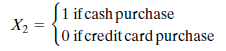and fit the regression model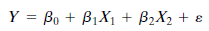c. Analyze the fit in part b. Be sure to include an analysis of the residuals. Are you happy with your model? d. Using the fitted model from part b, generate a forecast of daily sales for an individual that purchases 25 items and pays cash. Construct a large-sample 95% prediction interval for daily sales. e. Describe the nature of the fitted function in part b. Do you think it is better to fit two separate straight lines, one for the cash sales and another for the credit card sales, to the data in Table P-15? Discuss. TABLE P-15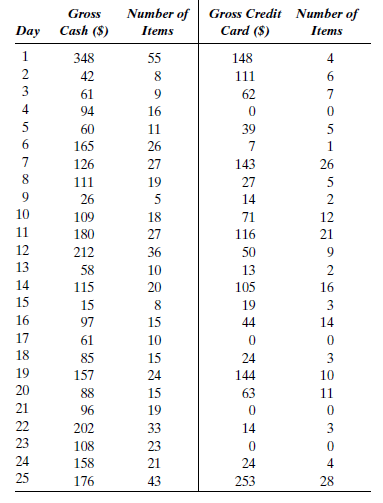Essay
TagsHaight, a real estate broker, wishes to forecast the importance of four factors in determining the prices of lots. She accumulates data on price, area, elevation, and slope and rates the view for 50 lots. She runs the data on a correlation program and obtains the correlation matrix given in Table P-17. Ms. Haight then runs the data on a stepwise multiple regression program. a. Determine which variable will enter the model first, second, third, and last. b. Which variable or variables will be included in the best prediction equation? TABLE P-17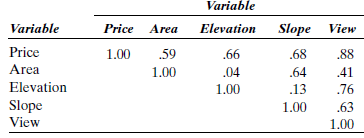Essay
TagsThe scores for two within-term examinations, X 1 and X 2 ; the current grade point average (GPA), X 3 ; and the final exam score, Y , for 20 students in a business statistics class are listed in Table P-18. a. Fit a multiple regression model to predict the final exam score from the scores on the within-term exams and GPA. Is the regression significant? Explain. b. Predict the final exam score for a student with within-term exam scores of 86 and 77 and a GPA of 3.4. c. Compute the VIF s and examine the t statistics for checking the significance of the individual predictor variables. Is multicollinearity a problem? Explain. d. Compute the mean leverage. Are any of the observations high leverage points? e. Compute the standardized residuals. Identify any observation with a large standardized residual. Does the fitted model under- or overpredict the response for these observations? TABLE P-18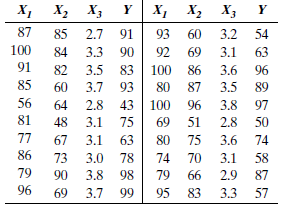Essay
TagsRefer to the data in Table P-18. Find the "best" regression model using the stepwise regression procedure and the all possible regressions procedure. Compare the results. Are you confident using a regression model to predict the final exam score with fewer than the original three independent variables?
EssayTable P-21 contains the number of accounts (in thousands) and the assets (in billions of dollars) for 10 online stock brokerages. Plot the assets versus the number of accounts. Investigate the possibility the relationship is curved by running a multiple regression to forecast assets using the number of accounts and the square of the number of accounts as independent variables. a. Give the fitted regression function. Is the regression significant? Explain. b. Test for the significance of the coefficient of the squared term. Summarize your conclusion. c. Rerun the analysis without the quadratic (squared) term. Explain why the coefficient of the number of accounts is not the same as the one you found for part a. TABLE P-21